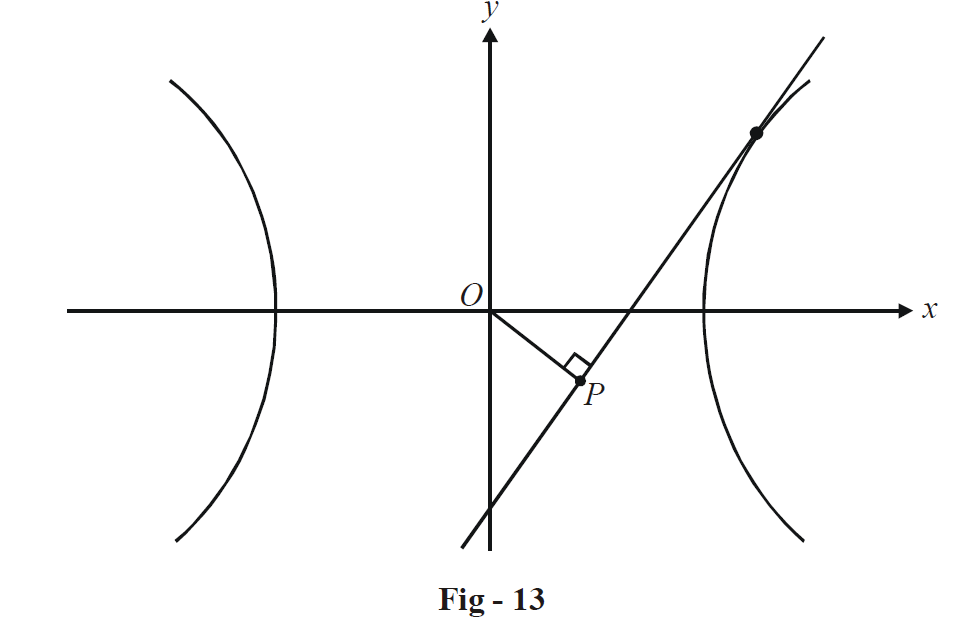# Examples on Tangents to Hyperbolas Set 2

Go back to  'Hyperbola'

Example - 14

Chords of the circle $${x^2} + {y^2} = {a^2}$$ touch the hyperbola \begin{align}\frac{{{x^2}}}{{{a^2}}} - \frac{{{y^2}}}{{{b^2}}} = 1.\end{align} Find the locus of the mid-points of such chords.

Solution : Assume the coordinates of the mid-point P as (h, k); we wish to determine the locus of P.

A chord of the circle $${x^2} + {y^2} = {a^2}$$ bisected at the point P has the equation

\begin{align}&\qquad \quad \;\;\;\;T(h,\,\,k) = S(h,\,\,k) \\\\ &\Rightarrow \qquad hx + ky - {a^2} = {h^2} + {k^2} - {a^2} \\\\&\Rightarrow \qquad y = \left( { - \frac{h}{k}} \right)x + \left( {\frac{{{h^2} + {k^2}}}{k}} \right) \\ \end{align}

This is a tangent to the given hyperbola if the condition for tangency $$({c^2} = {a^2}{m^2} - {b^2})$$  is satisfied, i.e. if

\begin{align}&\qquad\;\;\;\;\;{\left( {\frac{{{h^2} + {k^2}}}{k}} \right)^2} = {a^2}{\left( { - \frac{h}{k}} \right)^2} - {b^2} \\\\&\Rightarrow \qquad {({h^2} + {k^2})^2} = {a^2}{h^2} - {b^2}{k^2}\\\end{align}

Thus, we see that the locus of the mid point is

${({x^2} + {y^2})^2} = {a^2}{x^2} - {b^2}{y^2}$

Example - 15

From the center O of the hyperbola \begin{align}\frac{{{x^2}}}{{{a^2}}} - \frac{{{y^2}}}{{{b^2}}} = 1,\end{align} perpendicular OP is dropped upon any tangent to the hyperbola. Find the locus of P.

Solution :Assume the coordinates of P to be (h, k).

Any tangent to the given hyperbola has the form

$y = mx + \sqrt {{a^2}{m^2} - {b^2}}$

Since P lies on this tangent, we have

$k = mh + \sqrt {{a^2}{m^2} - {b^2}} \,\,\,\,\,\,\,\,\,\,\,\,\,\,\,\,\,\,\,\,\,\,\,\,\,\,\,\,\,\, \ldots \left( 1 \right)$

Also, since OP is perpendicular to this tangent, we have

\begin{align}&\qquad\;\;\frac{k}{h} \times m = - 1 \\ &\Rightarrow \quad m = - \frac{h}{k}\,\,\,\,\,\,\,\,\,\,\,\,\,\,\,\,\,\,\,\,\,\,\,\,\,\,\,\,\,\,\, \ldots \left( 2 \right)\\\end{align}

Using (2) in (1), we obtain a relation in h and k:

${({h^2} + {k^2})^2} = {a^2}{h^2} - {b^2}{k^2}$

Thus, the locus of P is

${({x^2} + {y^2})^2} = {a^2}{x^2} - {b^2}{y^2}$

## TRY YOURSELF - II

Q1. (a) Prove that the locus of the point of intersection of two perpendicular tangents to a hyperbola \begin{align}\frac{{{x^2}}}{{{a^2}}} - \frac{{{y^2}}}{{{b^2}}} = 1\end{align} will be the circle

${x^2} + {y^2} = {a^2} - {b^2}$

As for the other conics, such a circle is termed the Director circle of the hyperbola.

(b). Generalize the result of part (a). Prove that the locus of the point of intersection of two tangents to \begin{align}\frac{{{x^2}}}{{{a^2}}} - \frac{{{y^2}}}{{{b^2}}} = 1\end{align}  inclined at an angle $$\theta$$ will be

${({x^2} + {y^2} + {b^2} - {a^2})^2} = 4({a^2}{y^2} - {b^2}{x^2} + {a^2}{b^2}){\cot ^2}\theta$

Q2. Prove that the locus of the mid-point of the segment of a tangent to \begin{align}\frac{{{x^2}}}{{{a^2}}} - \frac{{{y^2}}}{{{b^2}}}\end{align} intercepted between the coordinate axes is

${a^2}{y^2} - {b^2}{x^2} = 4{x^2}{y^2}.$

Q3. Find the condition for the line $$x\cos \alpha + y\sin \alpha = p$$ to be a tangent to the hyperbola \begin{align}\frac{{{x^2}}}{{{a^2}}} - \frac{{{y^2}}}{{{b^2}}} = 1\end{align}.

Q4. Find the equations of the tangents to the hyperbola $$3{x^2} - {y^2} = 3$$ which are perpendicular to  $$x + 3y = 2.$$

Q5. Find the equation(s) of the common tangents to the parabola $${y^2} = 8x$$ and the hyperbola   $$3{x^2} - {y^2} = 3.$$

Download SOLVED Practice Questions of Examples on Tangents to Hyperbolas Set 2 for FREE
Hyperbolas
grade 11 | Questions Set 1
Hyperbolas
grade 11 | Answers Set 1
Hyperbolas
grade 11 | Questions Set 2
Hyperbolas
grade 11 | Answers Set 2
Learn from the best math teachers and top your exams

• Live one on one classroom and doubt clearing
• Practice worksheets in and after class for conceptual clarity
• Personalized curriculum to keep up with school